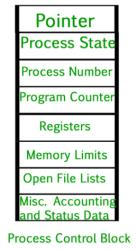Courses

# Test: Instrumentation & Process Control - 1

## 25 Questions MCQ Test Instrumentation and Process Control | Test: Instrumentation & Process Control - 1

Description
This mock test of Test: Instrumentation & Process Control - 1 for Electrical Engineering (EE) helps you for every Electrical Engineering (EE) entrance exam. This contains 25 Multiple Choice Questions for Electrical Engineering (EE) Test: Instrumentation & Process Control - 1 (mcq) to study with solutions a complete question bank. The solved questions answers in this Test: Instrumentation & Process Control - 1 quiz give you a good mix of easy questions and tough questions. Electrical Engineering (EE) students definitely take this Test: Instrumentation & Process Control - 1 exercise for a better result in the exam. You can find other Test: Instrumentation & Process Control - 1 extra questions, long questions & short questions for Electrical Engineering (EE) on EduRev as well by searching above.
QUESTION: 1

Solution:
QUESTION: 2

Solution:
QUESTION: 3

### Pick out the one which is a first order instrument.

Solution:
QUESTION: 4

Load cells are used for the measurement of

Solution:
QUESTION: 5

Which of the following is the dynamic characteristics of an instrument ?

Solution:
QUESTION: 6

Pick out the wrong statement.

Solution:
QUESTION: 7

The __________ of a vapor pressure thermometer is a functioning element.

Solution:
QUESTION: 8

A negative gain margin expressed in decibels means a/an __________ system.

Solution:
QUESTION: 9

Thermistor, which has high temperature co-efficient of resistivity, is used as the sensing element in resistance thermometer. It is a/an

Solution:
QUESTION: 10

Typical specifications for design stipulates the gain margin and phase margin to be respectively

Solution:
QUESTION: 11

Working principle of radiation pyrometer is based on the

Solution:
QUESTION: 12

Which of the following relates the emf. generated in a single homogeneous wire to the temperature difference ?

Solution:
QUESTION: 13

Dilute wine was used as a thermometric liquid initially to develop temperature scale. First emperical temperature scale developed was the __________ scale.

Solution:
QUESTION: 14

In a single tank system, the transfer function of level to inlet flow rate is

Solution:
QUESTION: 15

Which of the following is a desirable characteristic of an instrument ?

Solution:
QUESTION: 16

The operation of a rotameter is based on

Solution:
QUESTION: 17

Instrumentation in a plant offers the advantage of

Solution:
QUESTION: 18

Which of the following temperature measuring instruments need not touch the object whose temperature is being measured ?

Solution:
QUESTION: 19

The temperature of tempering oil baths maintained at 400oC during heat treatment of steel is measured by a/an __________ thermocouple.

Solution:
QUESTION: 20

Thermal conductivity measurement com-prises the working principle of a

Solution:
QUESTION: 21

Response of a system to a sinusoidal input is called __________ response.

Solution:
QUESTION: 22

The closed loop pole of a stable second order system could be

Solution:
QUESTION: 23

Smoke density of the flue gas going out of the chimney is measured by a

Solution:
QUESTION: 24

Transfer function of transportation lag is

Solution:
QUESTION: 25

Change of angle of refraction with composition comprises the working principle of a

Solution:

Track your progress, build streaks, highlight & save important lessons and more!

### Similar Content### Related tests